# Butterfly Delight

## Listed and emulated in MAME !
Info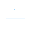(0)
Edit
Upload
### Butterfly Delight © 1996 Aristocrat.

A 5-reel video slot machine.
TECHNICAL INFORMATION
1
Aristocrat MKV hardware.

CPU : ARM250
Video Resolution : 640x400 pixels.
SCORING
All pays are left to right and/or right to left.

Girl appears on reel 3 and substitutes for all symbols.

Butterfly : 1 = 2, 2 = 20, 3 = 200, 4 = 1500, 5 = 7500
Bird : 1 = 2, 2 = 10, 3 = 100, 4 = 500, 5 = 5000
Bee : 2 = 5, 3 = 100, 4 = 300, 5 = 3000
Strawberry : 2 = 5, 3 = 100, 4 = 200, 5 = 2000
Blueberry : 2 = 5, 3 = 100, 4 = 200, 5 = 2000
Ace : 3 = 30, 4 = 100, 5 = 500
King : 3 = 30, 4 = 100, 5 = 500
Queen : 3 = 15, 4 = 100, 5 = 500
Jack : 3 = 15, 4 = 100, 5 = 500
Ten : 3 = 5, 4 = 50, 5 = 500
Nine : 3 = 5, 4 = 50, 5 = 500
Eight : 3 = 5, 4 = 50, 5 = 500
Flower : 3 = 2, 4 = 10, 5 = 50
TIPS AND TRICKS
Free Games Feature : 8 free games are won with 3, 4 or 5 scattered Flowers. Your overall win from the free games is repeated by the total Butterflies which appeared anywhere in the window during the free games. Additional free games can also be won during the feature.

Double Up Feature : At the end of a winning spin, or the last free game, you have the option of gambling the credits won. To double up, press the Gamble button and select Red or Black. You can exit the gamble feature by pressing Take Win even if you don't gamble the win. The double up feature will end if you lose, take the win or if you win 5 times in the same screen. If you win a jackpot at the same time as a game win, it is automatically added along with the jackpot and gamble is disabled for that spin.
SERIES
SOURCES
Game's artwork.
Game's ROMs.